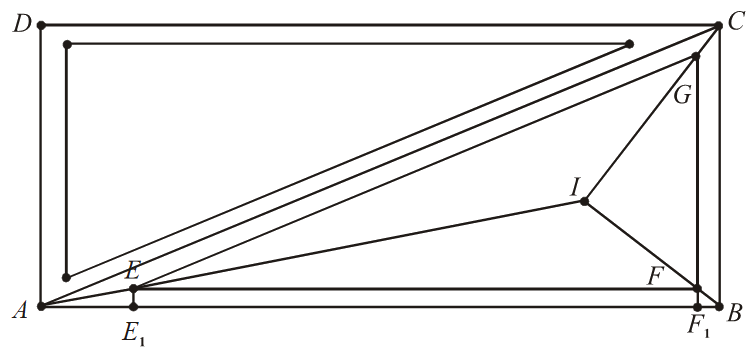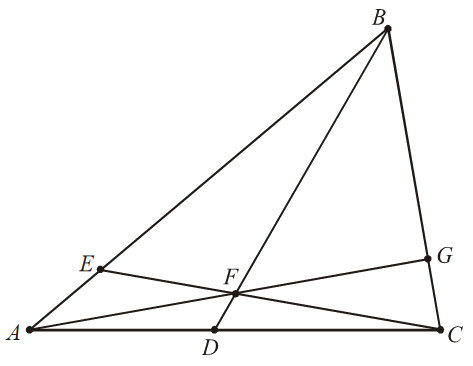# Trigonometry Set-5

Go back to  'SOLVED EXAMPLES'

Example - 11

A circle of radius 1 is randomly placed in a 15-by-36 rectangle $$ABCD$$ to that the circle lies completely within the rectangle. Given that the probability that the circle will not touch diagonal $$AC$$ is \begin{align}\frac{m}{n}\end{align}, where $$m$$ and $$n$$ are relatively prime positive integers, find $$m + n.$$

Solution: In order for the circle to lie completely within the rectangle, the center of the circle must lie in a rectangle that is (15 – 2) × (36 – 2), or 13 × 34. The requested probability is equal to the probability that the distance from the circle’s center to the diagonal $$AC$$ is greater than 1, which equals the probability that the distance from a randomly selected point in the 13 × 34 rectangle to each side of triangles $$ABC$$ and $$CDA$$ is greater than 1. Let | AB | = 36 and | BC | = 15 (and so | AC | = 39). Draw three segments that are 1 unit away from each side of triangle $$ABC$$ and whose endpoints are on the sides. Let $$E, F,$$ and $$G$$ be the three points of intersection nearest to $$A, B,$$ and $$C,$$ respectively, of the three segments. Because the corresponding sides of triangle $$ABC$$ and $$EFG$$ are parallel, the two triangles are similar to each other. The desired probability is equal to

\begin{align}\frac{{2\left[ {EFG} \right]}}{{13 \cdot 34}} = {\left( {\frac{{\left| {EF} \right|}}{{\left| {AB} \right|}}} \right)^2} \cdot \frac{{2\left[ {ABC} \right]}}{{13 \cdot 34}} = {\left( {\frac{{\left| {EF} \right|}}{{\left| {AB} \right|}}} \right)^2} \cdot \frac{{15 \cdot 36}}{{13 \cdot 34}} = {\left( {\frac{{\left| {EF} \right|}}{{\left| {AB} \right|}}} \right)^2} \cdot \frac{{270}}{{221}}.\end{align}

Because $$E$$ is equidistant from sides $$AB$$  and $$AC, E$$ lies on the bisector of $$CAB.$$ Similarly, $$F$$ and $$G$$ lie on the bisectors of \begin{align}\angle ABC\;{\rm{and}}\;\angle BCA\end{align}, respectively. Hence lines $$AE, BF,$$ and $$CG$$ meet $$I,$$ the incenter of triangle $$ABC.$$Let \begin{align}{E_1}\;{\rm{and}}\;{F_1}\end{align} be the feet of the perpendiculars from $$E$$ and $$F$$ to segment $$AB,$$ respectively. Then \begin{align}|EF| = |{E_1}{F_1}|\end{align}. It is not difficult to see that \begin{align}|B{F_1}| = |F{F_1}| = |E{E_1}| = 1\end{align}. Set \begin{align}\theta = \angle EAB\end{align}. Then \begin{align}\angle CAB = 2\theta ,\;\sin 2\theta = \frac{5}{{12}},\;\cos 2\theta = \frac{{12}}{{13}},\;{\rm{and}}\;2\theta = \frac{5}{{12}}\end{align}. By either the double-angle formulas or the half-angle formulas.

\begin{align}\tan 2\theta = \frac{{2\tan \theta }}{{1 - {{\tan }^2}\theta }}\;\;{\rm{or}}\;\;\tan \,\theta = \frac{{1 - \cos 2\theta }}{{\sin 2\theta }},\end{align}

and we obtain \begin{align}\tan \theta = \frac{1}{5}\end{align}. It follows that \begin{align}\left| {\frac{{E{E_1}}}{{A{E_1}}}} \right| = \tan \theta = \frac{1}{5},\;{\rm{or}}\;|A{E_1}| = 5\end{align}. Consequently, \begin{align}|EF|\; = \;|{E_1}\;{F_1}| = 30\end{align}. Hence \begin{align}\frac{m}{n} = {\left( {\frac{{30}}{{36}}} \right)^2} \cdot \frac{{270}}{{221}} = \frac{{375}}{{442}}\;{\rm{and}}\;m + n = 817.\end{align}

Example - 12

Evaluate

$\cos a\cos 2a\cos 3a.....\cos 999a$,

where \begin{align}a = \frac{{2\pi }}{{1999}}\end{align}.

Solution: Let P denote the desired product, and let

$Q = \sin a\sin 2a\sin 3a.....\sin 999a.$

Then

\begin{align} {2^{999}}PQ & = (2\sin a\cos a)(2\sin 2a\cos 2a)....(2\sin 999a\cos 999a)\\ & = \sin 2a\sin 4a....\sin 1998a\\ & = (\sin 2a\sin 4a...\sin 998a)[ - \sin (2\pi - 1000a)]\\ & \cdot \;[ - \sin (2\pi - 1002a)]...[ - \sin (2\pi - 1998a)]\\ & = \sin 2a\sin 4a...\sin 998a\sin 999a\sin 997a...\sin a = Q \end{align}

It is easy to see that \begin{align}Q \ne 0\end{align}. Hence the desired product is \begin{align}P = \frac{1}{{{2^{999}}}}.\end{align}

Example - 13

Let $$ABC$$ be a triangle with \begin{align}\angle BAC=40{}^\text{o}\ \text{and}\ \angle ABC=60{}^\text{o}\end{align}. Let $$D$$ and $$E$$ be the points lying on the sides $$AC$$ and $$AB,$$ respectively, such that \begin{align}\angle CBD=40{}^\text{o}\ \text{and}\ \angle BCE=70{}^\text{o}\end{align}. Segments $$BD$$ and $$CE$$ meet at $$F.$$ Show that \begin{align}AF\bot BC\end{align}.

Solution:Note that \begin{align}\angle ABD=20{}^\text{o},\ \angle BCA=80{}^\text{o},\ \text{and}\ \angle ACE=10{}^\text{o}.\end{align} Let $$G$$ be the foot of the altitude from $$A$$ to $$BC.$$ Then \begin{align}\angle BAG=90{}^\text{o}-\angle ABC=30{}^\text{o}\ \text{and}\ \angle CAG=90{}^\text{o}-\angle BCA=10{}^\text{o}\end{align}. Now,

\begin{align} \frac{\sin \angle BAG\sin \angle ACE\sin \angle CBD}{\sin \angle CAG\sin \angle BCE\sin \angle ABD} & =\frac{\sin 30{}^\text{o}\sin 10{}^\text{o}\sin 40{}^\text{o}}{\sin 10{}^\text{o}\sin 70{}^\text{o}\sin 20{}^\text{o}} \\ & =\frac{\frac{1}{2}(\sin 10{}^\text{o})(2\sin 20{}^\text{o}\cos 20{}^\text{o})}{\sin 10{}^\text{o}\cos 20{}^\text{o}\sin 20{}^\text{o}} \\ & =1. \\ \end{align}

Then by the trigonometric form of Ceva’s theorem, lines $$AB, BD$$ and $$CE$$ are concurrent. Therefore, $$F$$ lies on segment $$AG,$$ and so line $$AF$$ is perpendicular to the line $$BC,$$ as desired.

Learn from the best math teachers and top your exams

• Live one on one classroom and doubt clearing
• Practice worksheets in and after class for conceptual clarity
• Personalized curriculum to keep up with school